﻿ Filter Paper Salt Bridge in Potentiometric Titrations: An Undergraduate Laboratory Chemical Education Article

Filter Paper Salt Bridge in Potentiometric Titrations: An Undergraduate Laboratory Chemical Educatio...

World Journal of Chemical Education

Filter Paper Salt Bridge in Potentiometric Titrations: An Undergraduate Laboratory Chemical Education Article

G. Mallika Devi1, D. A. Padmavathi1, R. Sanjeev2, V. Jagannadham1,1Department of Chemistry, Osmania University, Hyderabad-500007, India

2Department of Chemistry, Geethanjali College of Engineering and Technology, Cheeryala, Rangareddy Dist., Telangana, India

Abstract

It is essential in an electrochemistry laboratory to use salt bridge in electrochemical cells while measuring the emf. This provides a means of eliminating “liquid junction potential” between two half cells. It was indicated laconically without any experimental details (https://en.wikipedia.org/wiki/Salt_bridge#cite_ref-1) that the salt bridges can also be made using laboratory filter paper soaking in a suitable inert salt solution. In this article we have made a detailed potentiometric experimental study on four representative titrations viz. strong acid (HCl) versus strong base (NaOH), weak acid (CH3COOH) versus strong base (NaOH), ferrous iron (Fe2+) versus dichromate (Cr2O72-), and chloride ion (Cl-) versus silver ion (Ag+) using filter paper salt bridge and compared the results with those obtained using Agar-Agar salt bridge.

• G. Mallika Devi, D. A. Padmavathi, R. Sanjeev, V. Jagannadham. Filter Paper Salt Bridge in Potentiometric Titrations: An Undergraduate Laboratory Chemical Education Article. World Journal of Chemical Education. Vol. 4, No. 6, 2016, pp 117-123. http://pubs.sciepub.com/wjce/4/6/1
• Devi, G. Mallika, et al. "Filter Paper Salt Bridge in Potentiometric Titrations: An Undergraduate Laboratory Chemical Education Article." World Journal of Chemical Education 4.6 (2016): 117-123.
• Devi, G. M. , Padmavathi, D. A. , Sanjeev, R. , & Jagannadham, V. (2016). Filter Paper Salt Bridge in Potentiometric Titrations: An Undergraduate Laboratory Chemical Education Article. World Journal of Chemical Education, 4(6), 117-123.
• Devi, G. Mallika, D. A. Padmavathi, R. Sanjeev, and V. Jagannadham. "Filter Paper Salt Bridge in Potentiometric Titrations: An Undergraduate Laboratory Chemical Education Article." World Journal of Chemical Education 4, no. 6 (2016): 117-123.

 Import into BibTeX Import into EndNote Import into RefMan Import into RefWorks

1. Introduction

In Daniell cell at the junction, the following situation is observed [1, 2]: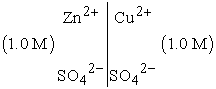If a small current is passed through the cell from left to right it is carried across the junction by Zn2+ ions and by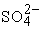ions. Similarly if a small current is passed from right to left it is carried across the junction by Cu2+ ions and byions. Thus the cell is not reversible. Reversibility can be applied only after the elimination of ‘liquid junction potential’. Generally this is successfully achieved by a device called ‘salt bridge’. Thus the cell is denoted as: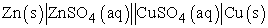A double vertical line || denotes an interface for which it is assumed that the ‘liquid junction potential’ is eliminated. Similarly a concentration cell is represented as: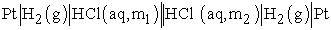It is needless to mention here about the details of reversibility of electrochemical cell as the aim of the present article is solely on electrochemical laboratory practice and on the emphasis of the construction and the successful use of ‘low cost filter paper salt bridge’. The conductivity depends on several factors: concentration of electrolyte solution, the consistency of pores of the filter paper and the other is the absorbing capacity of the filter paper. Usually a filter paper with soft texture and high absorbing capacity leads to higher conductivity. Therefore absolute measurements are not possible with filter paper salt bridge like estimating some standard physical properties: dissociation constants of weak acids (Ka), hydrolysis constants (Kh) of the salts of weak acid and strong base or weak base and a strong acid and solubility product (Ksp) of sparingly soluble salts in aqueous solution, yet the data obtained using low cost filter paper salt bridge is certainly useful in estimating the unknown concentrations of the solutions used.

The present article is an excellent practice in an undergraduate physical chemistry laboratory. This helps the students to record the potentiometric titrations experimental data, construction of figures in different ways viz. sigmoid plots, first and second derivative plots and interpretation of the final results.

2. Experimental

A detailed procedure for construction of a salt bridge is available in literature [3, 4, 5]. A low cost filter paper salt bridge could be made as follows: First we need a U-shaped glass tube. This could be made in a glass blowing work shop . Even a U-shaped cool-drink straw will do its job perfectly! Now a filter paper could be rolled as shown in Figure 1.

The rolled filter paper could be slowly insinuated in to the U-shaped glass tube or U-shaped cool-drink straw as shown in Figure 2.Download asVeiw figureFigures index
Figure 1.Download asVeiw figureFigures index
Figure 2.

All the required stock solutions were prepared using laboratory grade chemicals in the distilled water. All the figures were done using KaleidaGraph software version 4.1, supplied by Synergy Software, Reading, PA, USA.

3. Results and Discussion

Acid base titrations were carried out using quinhydrone electrode. The redox titration was carried out using platinum electrode. The precipitation titration was carried out using silver electrode. In the entire three titrations standard calomel electrode was used as the reference electrode.

The rolled filter paper inserted in to U-shaped tube is placed in a suitable salt solution of specified concentration usually of KCl or KNO3. The reason for choosing KCl or KNO3 is that the transport numbers of both cation and anion of the salts are almost same so that they carry same amount of current [1, 7]. The rolled filter paper will now be wetted with the salt solution as shown in Figure 3.Download asVeiw figureFigures index
Figure 3.

Figure 4 shows a typical potentiometric experimental set-up.Download asVeiw figureFigures index
Figure 4.

No gelification agent is required because the filter paper itself serves as medium of conduction. This can be used as salt bridge. U-shaped glass tube or U-shaped cool-drink straw is meant for giving the mechanical strength to the wetted rolled filter paper. Otherwise each time a new rolled filter paper has to be used for every new titration. This devise exactly replaces the Agar-Agar salt bridge constructed using salt solution gellified with Agar-Agar. The preparation of the Agar-Agar salt bridge is time consuming, tedious and little expensive. It needs Agar-Agar, usage of gas burner, and quite bit of the suitable salt either KCl or KNO3. Whereas the filter paper salt bridge needs a piece of filter paper and 10-15 ml of dilute salt solution. Thus the zero or filter paper salt bridge reduces the cost and saves the time. The titration of a strong acid (HCl, 0.1 M) with strong base (NaOH, 0.1 M), weak acid (CH3COOH, 0.1 M) with strong base (NaOH, 0.1 M), titration of ferrous iron (Fe2+, 0.1 M) with dichromate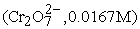and titration of chloride (Cl-, 0.05 M) with silver ion (Ag+, 0.05 M) were performed using the Agar-Agar salt bridge and zero or filter paper salt bridge. The results were tabulated in Table 1 and Table 2 and explained by suitable Figure 5-Figure 16.Download asVeiw figureFigures index
Figure 5A. Plot of the titration of strong acid (HCl= 0.1M) with strong base (NaOH=0.1M)Download asVeiw figureFigures index
Figure 5B. Plot of the titration of strong acid (HCl= 0.1M) with strong base (NaOH=0.1M)Download asVeiw figureFigures index
Figure 6. First derivative plot of the titration of strong acid (HCl= 0.1M) with strong base (NaOH=0.1M)Download asVeiw figureFigures index
Figure 7A. Second derivative plot of the titration of strong acid (HCl= 0.1M) with strong base (NaOH=0.1M)Download asVeiw figureFigures index
Figure 7B. Second derivative plot of the titration of strong acid (HCl= 0.1M) with strong base (NaOH=0.1M)Download asVeiw figureFigures index
Figure 8A. Plot of the titration of weak acid (CH3COOH= 0.1M) with strong base (NaOH=0.1M)Download asVeiw figureFigures index
Figure 8B. Plot of the titration of weak acid (CH3COOH= 0.1M) with strong base (NaOH=0.1M)Download asVeiw figureFigures index
Figure 9. First derivative plot of the titration of weak acid (CH3COOH= 0.1M) with strong base (NaOH=0.1M)Download asVeiw figureFigures index
Figure 10A. Second derivative plot of the titration of weak acid (CH3COOH= 0.1M) with strong base (NaOH=0.1M)Download asVeiw figureFigures index
Figure 10B. Second derivative plot of the titration of weak acid (CH3COOH= 0.1M) with strong base (NaOH=0.1M)Download asVeiw figureFigures index
Figure 11A. Plot of the titration of Ferrous Iron (Fe2+=0.1M) with dichromate (Cr2O72-=0.067M)Download asVeiw figureFigures index
Figure 11B. Plot of the titration of Ferrous Iron (Fe2+=0.1M) with dichromate (Cr2O72-=0.067M)Download asVeiw figureFigures index
Figure 12. First derivative plot of the titration of Ferrous Iron (Fe2+=0.1M) with dichromate (Cr2O72-=0.067M)Download asVeiw figureFigures index
Figure 13. First derivative plot of the titration of Ferrous Iron (Fe2+=0.1M) with dichromate (Cr2O72-=0.067M)Download asVeiw figureFigures index
Figure 14A. Plot of the titration of chloride ion (0.05M) with Ag+ (0.05M)Download asVeiw figureFigures index
Figure 14B. Plot of the titration of chloride ion (0.05M) with Ag+ (0.05M)Download asVeiw figureFigures index
Figure 15. First derivative plot of the titration of chloride ion (0.05M) with Ag+ (0.05M)Download asVeiw figureFigures index
Figure 16. Second derivative plot of the titration of chloride ion (0.05M) with Ag+ (0.05M)

Results of the titration of strong acid vs strong base with Agar-Agar salt bridge and zero or filter paper salt bridge is given Table 1 and Figure 5, Figure 6 and Figure 7. Results of the titration of weak acid vs strong base with Agar-Agar salt bridge and zero or filter paper salt bridge are given in Table 1 and Figure 8, Figure 9 and Figure 10. Results of the titration of Fe2+ vs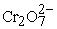with Agar-Agar salt bridge and zero or filter paper salt bridge is given in Table 2 and Figure 11, Figure 12 and Figure 13. Results of the titration of Cl- vs Ag+ with Agar-Agar salt bridge and zero or filter paper salt bridge (soaked in KNO3 solution) is given in Table 2 and Figure 14, Figure 15 and Figure 16. Figure 5, Figure 8, Figure 11 and Figure 14 show that though the absolute potentials differ to a smaller extent in both the measurements using the two salt bridges, yet the trend in the equivalence point are unmistakable. Figure 6, Figure 9, Figure 12 and Figure 15 show the equivalence points from 1st derivative plots. Similarly Figure 7, Figure 10, Figure 13 and Figure 16 show the equivalence points from 2nd derivative plots.

The final results are given in Table 3. The results are compared and found promising within 3-6 % experimental error except in the case of precipitation titration explains the importance and advantage of zero or filter paper salt bridge. Even that 10% error in the precipitation titration is only from sigmoid plot Figure 14B. Table 3 column-2 gives the equivalence points from direct titration using chemical method with suitable indicators. Figure 5B is an expanded version of the Figure 5A. Approximately two parallel lines AB and CD were drawn in the beginning and at the end of the titration in Figure 5B. A parallel line EF to X-axis is drawn midway between AB and CD. Another two perpendicular lines GH and IJ were drawn parallel to y-axis. At the points of intersection of EF with GH and IJ gives the equivalence points of the titration. Similarly Figure 8B, Figure 11B and Figure 14B were constructed. The results were tabulated in table-3. Figure 7B is an expanded version of the Figure 7A on X-axis in order to see the equivalence point clearly on the second derivative plot. Similarly the Figure 10B is an expanded version of the Figure 10A. The equivalence points from double derivative plots are found from the points of intersection of the x-axis and the straight locus joining the two points which are away at a maximum distance from the X-axis.

4. Conclusions

The present work provides a comprehensive study of potentiometric titrations, plotting the results and their interpretation to an undergraduate physical chemistry laboratory class-room. Instead of a glass tube a U-shaped ordinary plastic tube will work perfectly. Even one can use a U-shaped cool-drink straw available in bakeries for this purpose! The use and practice of KaleidaGraph (Synergy Software, Reading, PA, USA) is very user friendly to undergraduate students and easily practicable, hence advised to buy for every college/university.

References

  W. J. Moore, Physical Chemistry, Orient Longmans, Copy right 1962 by Printice Hall Inc., Englewood Cliffs, New Jersey, USA.In article  P. W. Atkins, Physical Chemistry, Oxford University Press, 5th Edition, Oxford, Melbourne, Tokyo, 1994.In article  B.P. Levitt, Findlays Practical Physical Chemistry (9th. Ed.) Longman Group Ltd. 1973.In article  Hogendoorn, Bob (2010). Heinemann Chemistry Enhanced (2). Melbourne, Australia: Pearson Australia. p. 416.In article  https://www.google.co.in/?gfe_rd=cr&ei=dAziVISUFsPM8geU7oDoCA&gws_rd=ssl#q=preparation+of+salt+bridge.In article  https://www.youtube.com/watch?v=wk8jyXZ2oGw.In article  L. G. Longsworth, J. Am. Chem. Soc., 57, 1185 (1935) and 60, 3070 (1938).In article